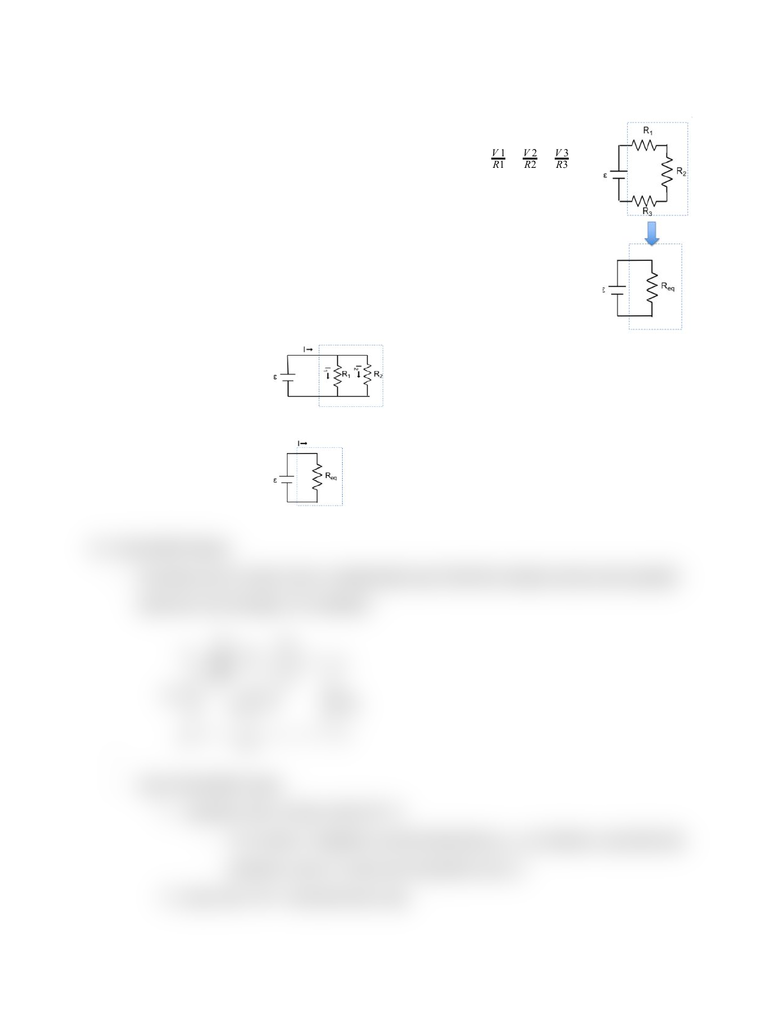Get 2 days of premium access
Class Notes (1,000,000)
US (430,000)
UC-Irvine (10,000)
PHYSICS (500)
Lecture 13

# PHYSICS 7D Lecture Notes - Lecture 13: American Broadcasting CompanyPremium

Department
Physics
Course Code
PHYSICS 7D
Professor
William Molzon
Lecture
13

This preview shows half of the first page. to view the full 2 pages of the document.Physics 7C Lecture 13: Direct-Current Circuits
I. Resistors in Series
- Current is the same through all resistors: I = = =
R1
V1
R2
V2
R3
V3
- Total voltage drop must = ε
- ε= IR1 +IR2+IR3= I(R1+R2+R3)
- ε=IReq
- => Req=R1+R2+R3
so Req,= ΣR
II. Resistors in Parallel
- 1/Req= Σ (1/RI)
III. Kirchhoff's Rules
- Circuits can be much more complicated such that the simple series and parallel
rules are not enough, for example:
-
- Use Kirchhoff's rules:
1. Junction rule: at any node ΣI= 0
- For node a: Assume current directions i3-i1-i2=0 where currents into
junction a are (+) and out of junction are (-)
2. Loop rule: ΣV= 0 around any loop
###### You're Reading a Preview

Unlock to view full version

Subscribers Only